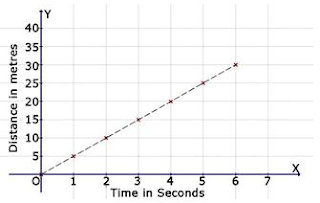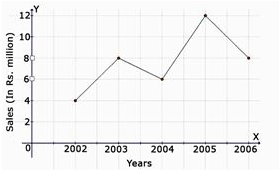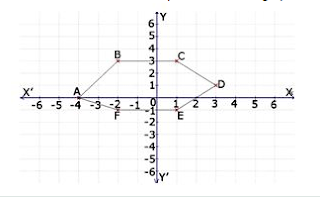# MCQ Questions for Class 8 Maths Chapter 15 Introduction to Graphs with Answers

Students can access the NCERT MCQ Questions for Class 8 Maths Chapter 15 Introduction to Graphs with Answers Pdf free download aids in your exam preparation and you can get a good hold of the chapter. Use MCQ Questions for Class 8 Maths with Answers during preparation and score maximum marks in the exam. Students can download the Introduction to Graphs Class 8 MCQs Questions with Answers from here and test their problem-solving skills. Clear all the fundamentals and prepare thoroughly for the exam taking help from Class 8 Maths Chapter 15 Introduction to Graphs Objective Questions.

## Introduction to Graphs Class 8 MCQs Questions with Answers

Students are advised to solve the Introduction to Graphs Multiple Choice Questions of Class 8 Maths to know different concepts. Practicing the MCQ Questions on Introduction to Graphs Class 8 with answers will boost your confidence thereby helping you score well in the exam.

Explore numerous MCQ Questions of Introduction to Graphs Class 8 with answers provided with detailed solutions by looking below.

Question 1.
Find the coordinates of the point A from the graph.
(a) (-4, 5)
(b) (-4, 0)
(c) (0, -4)
(d) none of these

Question 2.
Which of the following statements is true?
(a) The X-axis is a vertical line.
(b) The Y-axis is a horizontal line.
(c) The scale on both the axes must be the same in a Cartesian plane.
(d) The point of intersection between the X-axis and Y-axis is called the origin.

Answer: (d) The point of intersection between the X-axis and Y-axis is called the origin.

Question 3.
The line graph shows the yearly sales figure for a manufacturing company. From the graph, what were the sales in 2006?
(a) Rs 8 millions
(b) Rs 4 millions
(c) Rs 3 millions
(d) Rs 5 millions

Question 4.
In which quadrant does the point Q (-2, -6) lie?
(a) I
(b) II
(c) IV
(d) III

Question 5.
Which of the points given is a point on the X-axis?
(a) (5,0)
(b) (0,5)
(c) (5,3)
(d) (3,5)

Question 6.
Find the distance covered in 2 seconds.(a) 15 m
(b) 20 m
(c) 25 m
(d) 10 m

Question 7.
Find the coordinates of the point E from the graph.(a) (1, -1)
(b) (-4, 5)
(c) (0, -4)
(d) None of these

Question 8.
Find the distance covered in 6 seconds.(a) 30 m
(b) 35 m
(c) 25 m
(d) none of these

Question 9.
Find the distance covered in 7 seconds.(a) 30 m
(b) 25 m
(c) 35 m
(d) 20 m

Question 10.
Find the time taken by a body to cover 5 metres.(a) 3 seconds
(b) 4 seconds
(c) 5 seconds
(d) 1 second

Question 11.
The line graph shows the yearly sales figure for a manufacturing company. From the graph, what were the sales in 2004?(a) Rs 5 millions
(b) Rs 3 millions
(c) Rs 4 millions
(d) Rs 6 millions

Question 12.
On which axis does the point (-1, 0) lie?
(a) x-axis
(b) origin
(c) y-axis
(d) None of these

Question 13.
Find the coordinates of the point C from the graph.(a) (1, 3)
(b) (-4, 5)
(c) (0, -4)
(d) None of these

Question 14.
On which axis does the point (0, 5) lie?
(a) origin
(b) x-axis
(c) y-axis
(d) None of these

Question 15.
Find the coordinates of the point F from the graph.(a) (-4, 5)
(b) (-2, -1)
(c) (0, -4)
(d) None of these

Question 16.
Find the distance covered in 5 seconds.(a) 25 m
(b) 10 m
(c) 20 m
(d) none of these